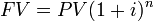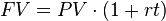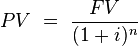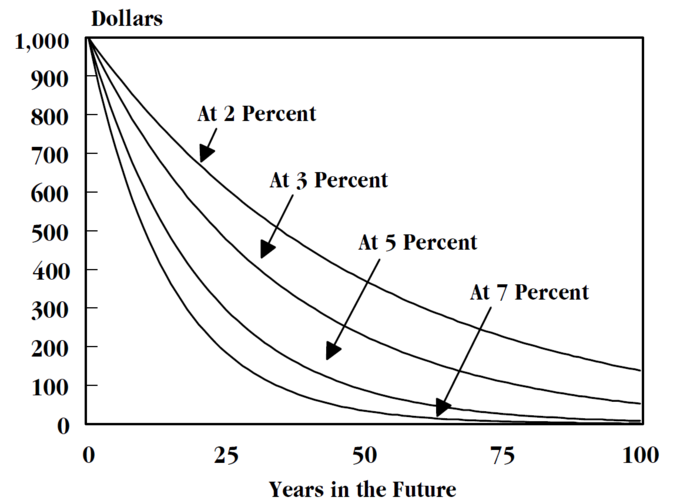## The Discount Rate

Discounting is the procedure of finding what a future sum of money is worth today.

### Learning Objectives

Describe what real world costs to the investor comprise an investment’s interest rate

### Key Takeaways

#### Key Points

• The discount rate represents some cost (or group of costs) to the investor or creditor.
• Some costs to the investor or creditor are opportunity cost, liquidity cost, risk, and inflation.
• The discount rate is used by both the creditor and debtor to find the present value of an amount of money.

#### Key Terms

• discount rate: The interest rate used to discount future cash flows of a financial instrument; the annual interest rate used to decrease the amounts of future cash flow to yield their present value.
• discounting: The process of finding the present value using the discount rate.
• discount: to account for the time value of money

Another common name for finding present value (PV) is discounting . Discounting is the procedure of finding what a future sum of money is worth today. As you know from the previous sections, to find the PV of a payment you need to know the future value (FV), the number of time periods in question, and the interest rate. The interest rate, in this context, is more commonly called the discount rate.

The discount rate represents some cost (or group of costs) to the investor or creditor. The sum of these costs amounts to a percentage which becomes the interest rate (plus a small profit, sometimes). Here are some of the most significant costs from the investor/creditor’s point of view:Borrowing and lending: Banks like HSBC take such costs into account when determining the terms of a loan for borrowers.

1. Opportunity Cost: The cost of not having the cash on hand at a certain point of time. If the investor/creditor had the cash s/he could spend it, but since it has been invested/loaned out, s/he incurs the cost of not being able to spend it.
2. Inflation: The real value of a single dollar decreases over time with inflation. That means that even if everything else is constant, a $100 item will retail for more than$100 in the future. Inflation is generally positive in most countries at most times (if it’s not, it’s called deflation, but it’s rare).
3. Risk: There is a chance that you will not get your money back because it is a bad investment, the debtor defaults. You require compensation for taking on that risk.
4. Liquidity: Investing or loaning out cash necessarily reduces your liquidity.

All of these costs combine to determine the interest rate on an account, and that interest rate in turn is the rate at which the sum is discounted.

The PV and the discount rate are related through the same formula we have been using, $\frac { FV }{ [(1+i)]^n }$.

If FV and n are held as constants, then as the discount rate (i) increases, PV decreases. PV and the discount rate, therefore, vary inversely, a fundamental relationship in finance. Suppose you expect $1,000 dollars in one year’s time (FV =$1,000). To determine the present value, you would need to discount it by some interest rate (i). If this discount rate were 5%, the $1,000 in a year’s time would be the equivalent of$952.38 to you today (1000/[1.00 + 0.05]).

## Number of Periods

The number of periods corresponds to the number of times the interest is accrued.

### Learning Objectives

Define what a period is in terms of present value calculations

### Key Takeaways

#### Key Points

• A period is just a general term for a length of time. It can be anything- one month, one year, one decade- but it must be clearly defined and fixed.
• For both simple and compound interest, the number of periods varies jointly with FV and inversely with PV.
• The number of periods is also part of the units of the discount rate: if one period is one year, the discount rate must be defined as X% per year. If one period is one month, the discount rate must be X% per month.

#### Key Terms

• period: The length of time during which interest accrues.

In, nrepresents the number of periods. A period is just a general term for a length of time. It can be anything- one month, one year, one decade- but it must be clearly defined and fixed. The length of one period must be the same at the beginning of an investment and at the end. It is also part of the units of the discount rate: if one period is one year, the discount rate must be defined as X% per year. If one period is one month, the discount rate must be X% per month.FV of a single payment: The PV and FV are directly related.

The number of periods corresponds to the number of times the interest is accrued. In the case of simple interest the number of periods, t, is multiplied by their interest rate. This makes sense because if you earn $30 of interest in the first period, you also earn$30 of interest in the last period, so the total amount of interest earned is simple t x \$30.

Simple interest is rarely used in comparison to compound interest. In compound interest, the interest in one period is also paid on all interest accrued in previous periods. Therefore, there is an exponential relationship between PV and FV, which is reflected in (1+i)n .Car: Car loans, mortgages, and student loans all generally have compound interest.

For both forms of interest, the number of periods varies jointly with FV and inversely with PV. Logically, if more time passes between the present and the future, the FV must be higher or the PV lower (assuming the discount rate remains constant).

## Calculating Present Value

Calculating the present value (PV) is a matter of plugging FV, the interest rate, and the number of periods into an equation.

### Learning Objectives

Distinguish between the formula used for calculating present value with simple interest and the formula used for present value with compound interest

### Key Takeaways

#### Key Points

• The first step is to identify if the interest is simple or compound. Most of the time, it is compound.
• The interest rate and number of periods must have consistent units.
• The PV is what a future sum is worth today given a specific interest rate (often called a “discount rate”).

#### Key Terms

• compound interest: Interest, as on a loan or a bank account, that is calculated on the total on the principal plus accumulated unpaid interest.
• simple interest: interest paid only on the principal.

Finding the present value (PV) of an amount of money is finding the amount of money today that is worth the same as an amount of money in the future, given a certain interest rate.

Calculating the present value (PV) of a single amount is a matter of combining all of the different parts we have already discussed. But first, you must determine whether the type of interest is simple or compound interest. If the interest is simple interest, you plug the numbers into the simple interest formula.Simple Interest Formula: Simple interest is when interest is only paid on the amount you originally invested (the principal). You don’t earn interest on interest you previously earned.

If it is compound interest, you can rearrange the compound interest formula to calculate the present value.Present Value Single Payment: Finding the PV is a matter of plugging in for the three other variables.

### Inputs

• Future Value: The known value of the money at a declared point in the future.
• Interest Rate (Discount Rate): Represented as either i or r. This is the percentage of interest paid each period.
• Number of periods: Represented as n or t.

Once you know these three variables, you can plug them into the appropriate equation. If the problem doesn’t say otherwise, it’s safe to assume the interest compounds. If you happen to be using a program like Excel, the interest is compounded in the PV formula. Simple interest is pretty rare.

One area where there is often a mistake is in defining the number of periods and the interest rate. They have to have consistent units, which may require some work. For example, interest is often listed as X% per year. The problem may talk about finding the PV 24 months before the FV, but the number of periods must be in years since the interest rate is listed per year. Therefore, n = 2. As long as the units are consistent, however, finding the PV is done by plug-and-chug.

## Multi-Period Investment

Multi-period investments require an understanding of compound interest, incorporating the time value of money over time.

### Learning Objectives

Calculate the return on a multi-period investment over time

### Key Takeaways

#### Key Points

• A dollar today is worth more than a dollar tomorrow, and the time value of money must take into account foregone opportunities.
• Single period investments are relatively simple to calculate in terms of future value, applying the interest rate to a present value a single time.
• Multi-period investments require a slightly more complex equation, where interest gets compounded based on the number of periods the investment spans.
• As a result of multiple periods, it is usually a good idea to calculate the average rate of return (cumulatively) over the lifetime of the investment.

#### Key Terms

• Compound interest: An interest rate applied to multiple applications of interest during the lifetime of the investment.

When investing, the time value of money is a core concept investors simply cannot ignore. A dollar today is valued higher than a dollar tomorrow, and when utilizing the capital it is important to recognize the opportunity cost involved in what could have been invested in instead.

### Single Period Investments

With single period investments, the concept of time value of money is relatively straightforward. The future value is simply the present value applied to the interest rate compounded one time. When comparing this to the opportunity costs involved, the rate of return of an alternative investment during the same time is similarly straightforward.

The variables involved in understanding the time value of money in these investments are:

• Present Value (PV)
• Future Value (FV)
• Interest Rate (i or r) [Note: for all formulas, express interest in its decimal form, not as a whole number. 7% is.07, 12% is.12, and so on. ]
• Number of Periods (t or n)

With these variables, a single period investment could be calculated as follows:

$FV=PV(1+rt)$

‘t’ in this equation would simply be 1, simplifying this equation to FV = PV(1+r).

### Multi-period Investments

With multi-periods in mind, interest begins to compound. Compound interest simple means that the interest from the first period is added to the future present value, and the interest rate the next time around is now being applied to a larger amount. This turns into an exponential calculation of interest, calculated as follows:

$FV=PV(1+i)^{t}$

This means that the interest rate of a given period may not be the same percentage as the interest rate over multiple periods (in most situations). A useful tool at this point is a way to create an average rate of return over the life of the investment, which can be derived with the following:

${\bar {r}}={\frac {1}{n}}\sum _{i=1}^{n}{r_{i}}={\frac {1}{n}}(r_{1}+\cdots +r_{n})$

### Conclusion

All and all, the difference from a time value of money perspective between single and multiple period investments is relatively straightforward. Normalizing expected returns in present value terms (or projecting future returns over multiple time periods of compounding interest) paints a clearer and more accurate picture of the actual worth of a given investment opportunity.Time Value of Money: Time value of money requires an understanding of how return rates impact fixed values over time.

## Single-Period Investment

When considering a single-period investment, n is one, so the PV is simply FV divided by 1+i.

### Learning Objectives

Calculate the present value of a future, single-period payment

### Key Takeaways

#### Key Points

• A single period investment has the number of periods (n or t) equal to one.
• For both simple and compound interest, the PV is FV divided by 1+i.
• The time value of money framework says that money in the future is not worth as much as money in the present.

#### Key Terms

• period: The length of time during which interest accrues.
• interest rate: The percentage of an amount of money charged for its use per some period of time. It can also be thought of as the cost of not having money for one period, or the amount paid on an investment per year.

The time value of money framework says that money in the future is not worth as much as money in the present. Investors would prefer to have the money today because then they are able to spend it, save it, or invest it right now instead of having to wait to be able to use it.

The difference between what the money is worth today and what it will be worth at a point in the future can be quantified. The value of the money today is called the present value (PV), and the value of the money in the future is called the future value (FV). There is also a name for the cost of not having the money today: the interest rate or discount rate (i or r). For example, if the interest rate is 3% per year, it means that you would be willing to pay 3% of the money to have it one year sooner. The amount of time is also represented by a variable: the number of periods (n). One period could be any length of time, such as one day, one month, or one year, but it must be clearly defined, consistent with the time units in the interest rate, and constant throughout your calculations.FV of a single payment: The FV is related to the PV by being i% more each period.

All of these variables are related through an equation that helps you find the PV of a single amount of money. That is, it tells you what a single payment is worth today, but not what a series of payments is worth today (that will come later). relates all of the variables together. In order to find the PV, you must know the FV, i, and n.

When considering a single-period investment, n is, by definition, one. That means that the PV is simply FV divided by 1+i. There is a cost to not having the money for one year, which is what the interest rate represents. Therefore, the PV is i% less than the FV.